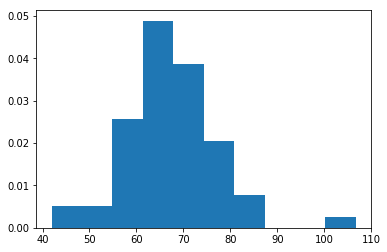# Z-Scores and Inferential Stats in Python

In this post, we will look at some ways to calculate some inferential statistics in Python. We are mainly going to focus on z-scores and one/two-tailed test.

We will begin by import some needed packages and then we will make some data and plot it.  Below is the code and plot

```import numpy as np
import matplotlib.pyplot as plt
import scipy.stats as stats
quizscore=np.random.normal(69,9,60)
plt.hist(quizscore,density=True)```We create an object called “quizscore” which contains as you can tell quiz scores. In line four in the code above, we used the .random.normal function from the numpy package to create some random data. This function has 3 arguments in it, the first is the mean of the distribution, next is the standard deviation,and the last is the sample size. After this is the code for the histogram which uses the .hist function from the matplotlib.pyplot package.

Z-Scores

Z-Scores are a measure of how far a data point is from the mean. In the code below, we calculate the first five z-scores of our “quizscore” dataset.

```stats.zscore(quizscore)[0:5,]
Out: array([ 0.54362001, 1.56135871, -0.36924596, 0.53182556, -0.06014972])```

In the output, you can see that the first number in the dataset is 0.54 standard deviations above the mean. Remember, our standard deviation was set to 9 so this means the score for the first quiz was about 73. We confirm this with the code below.

```quizscore[0,]
Out: 72.851341820695538```

Fairly close, another question we can answer is what is the probability that someone got a score that was 1.5 standard deviations or higher on the quiz (about a score of 82). Below is the code followed by the answer.

```1-stats.norm.cdf(1.5)
Out: 0.066807201268858085```

In the code above we subtract 1 from our code. The code uses the .cdf function. Inside the function, we put our z-score of 1.5. The answer is 0.066 or 6% of the scores have a Z-score of 1.5 or higher or a quiz score of around 82 or higher.

We can also determine what is the cutoff point for a certain high score. For us, we want to know what the cutoff for the top 15% of quiz scores. Below is the code.

```stats.norm.ppf(0.85)
Out: 1.0364333894937898

(1.03 * quizscore.std()) +quizscore.mean()
Out: 77.748927759179054```

In the code above, first we had to convert the percentage to a z-score and this is done with the .ppf function. The 85 percentile is equivalent to a Z-score of about 1. Next, we multiplied the z-score by the standard deviation of the quizscore and added the mean of the quizscore to this to get our final answer of 77.74. This means that a score of 77.74 and above is within the top 15%.

One & Two-Tailed Test

A one-tail test is used to compare the sample mean to a population mean. Another way to look at this is to convert a z-score to a p-value. What is happening here is you create a region in your distribution in which scores are considered unusual. For a one-tail test, this is the top 5%. For example, let’s say you want to know the probability that someone got a score of 92 or higher on the quiz. This can be solved with the following code.

```quizZ=(92-quizscore.mean())/quizscore.std()
1-stats.norm.cdf(quizZ)
Out: 0.0072370644085374414```

Here is what we did

1. We create an object called “quizZ” which took our specific score of 92 subtracted the mean of “quizscore” and divided it by the standard deviation of “quizscore”. This becomes the z-score for the value 92.
2. We then subtract one from this while using the .cdf function
3. The output indicates that there is less than a 1% chance that someone got a score of 92% or higher.

In this example our sample mean was small (only 1) but the concept remains the same.

A two-tailed test is the same concept except that the rejections regions are on both sides of the distribution and divide in half. This means that the top 2.5% and bottom 2.5% are now considered unusual scores. As an example, if the average score on the quiz is 75 with a standard deviation of 5 and we want to see if our class mean of 67 is different. We can use the following code to answer this.

```two_tail=(quizscore.mean()-75)/5
stats.norm.cdf(two_tail)
Out: 0.063688920253590395```

You can see that the probability of our class is not unusual as it is above the cutoff of 5% indicating no difference. This means that if 75 is the center of our distribution the quiz score of 67 would be within 2 standard deviations of the mean.

Conclusion

In this post, we explored some of the ways you can do inferential statistics with Python. Whatever you want to know can be calculated by knowing a few lines of code.

## 1 thought on “Z-Scores and Inferential Stats in Python”

1.Ken Popkin